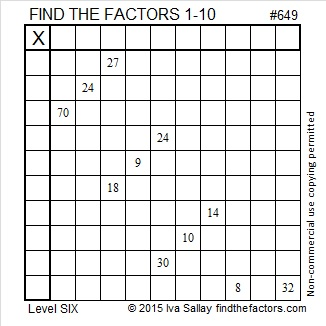# 649 and Level 6

6 – 4 + 9 = 11 so 649 is divisible by 11.

649 is the short leg in exactly three Pythagorean triples. Can you determine which one is a primitive triple, and what are the greatest common factors of each of the two non-primitive triples?

• 649-3540-3599
• 649-19140-19151
• 649-210600-210601Print the puzzles or type the solution on this excel file: 10 Factors 2015-10-12

—————————————————————————————————

• 649 is a composite number.
• Prime factorization: 649 = 11 x 59
• The exponents in the prime factorization are 1 and 1. Adding one to each and multiplying we get (1 + 1)(1 + 1) = 2 x 2 = 4. Therefore 649 has exactly 4 factors.
• Factors of 649: 1, 11, 59, 649
• Factor pairs: 649 = 1 x 649 or 11 x 59
• 649 has no square factors that allow its square root to be simplified. √649 ≈ 25.475478.—————————————————————————————————